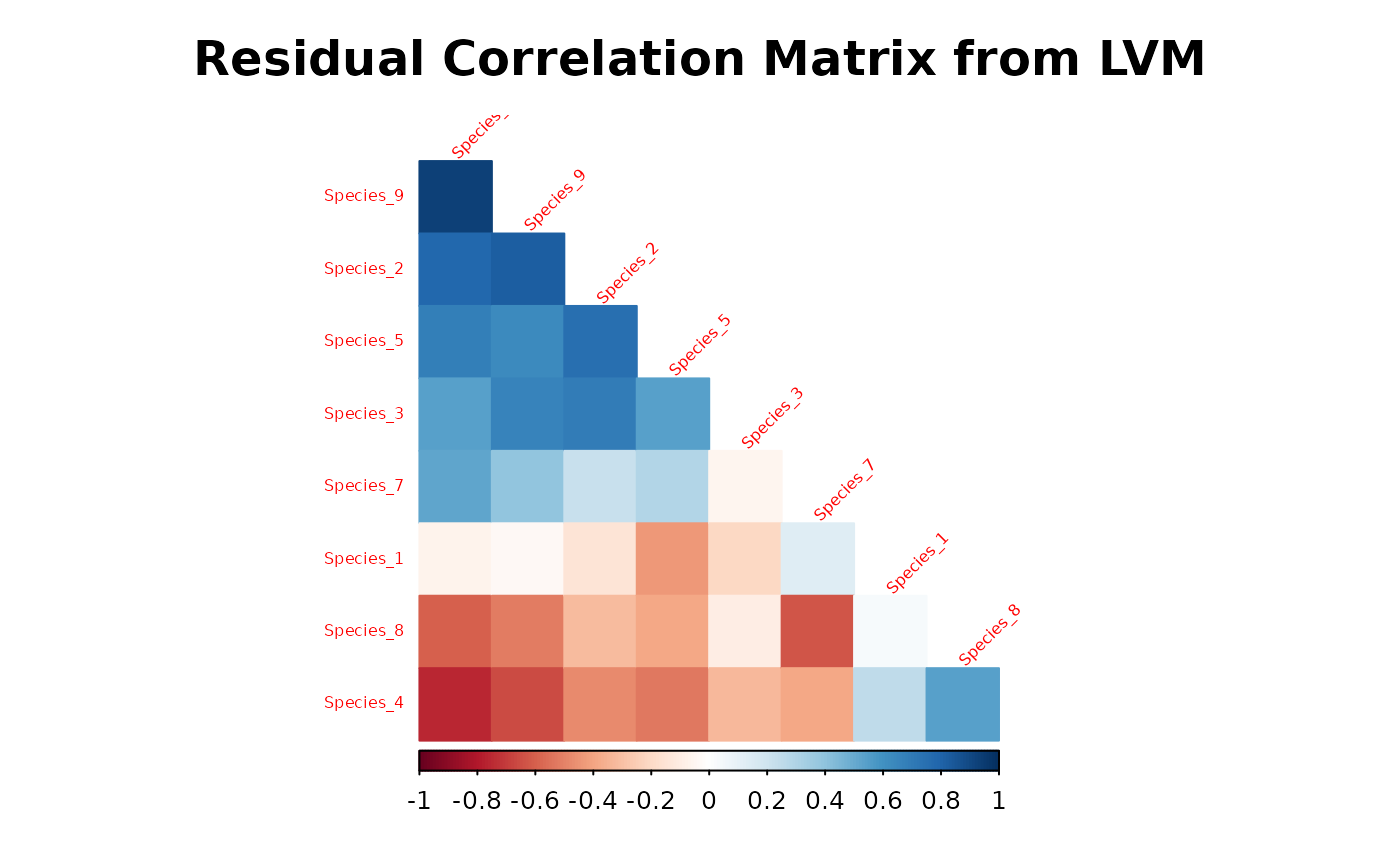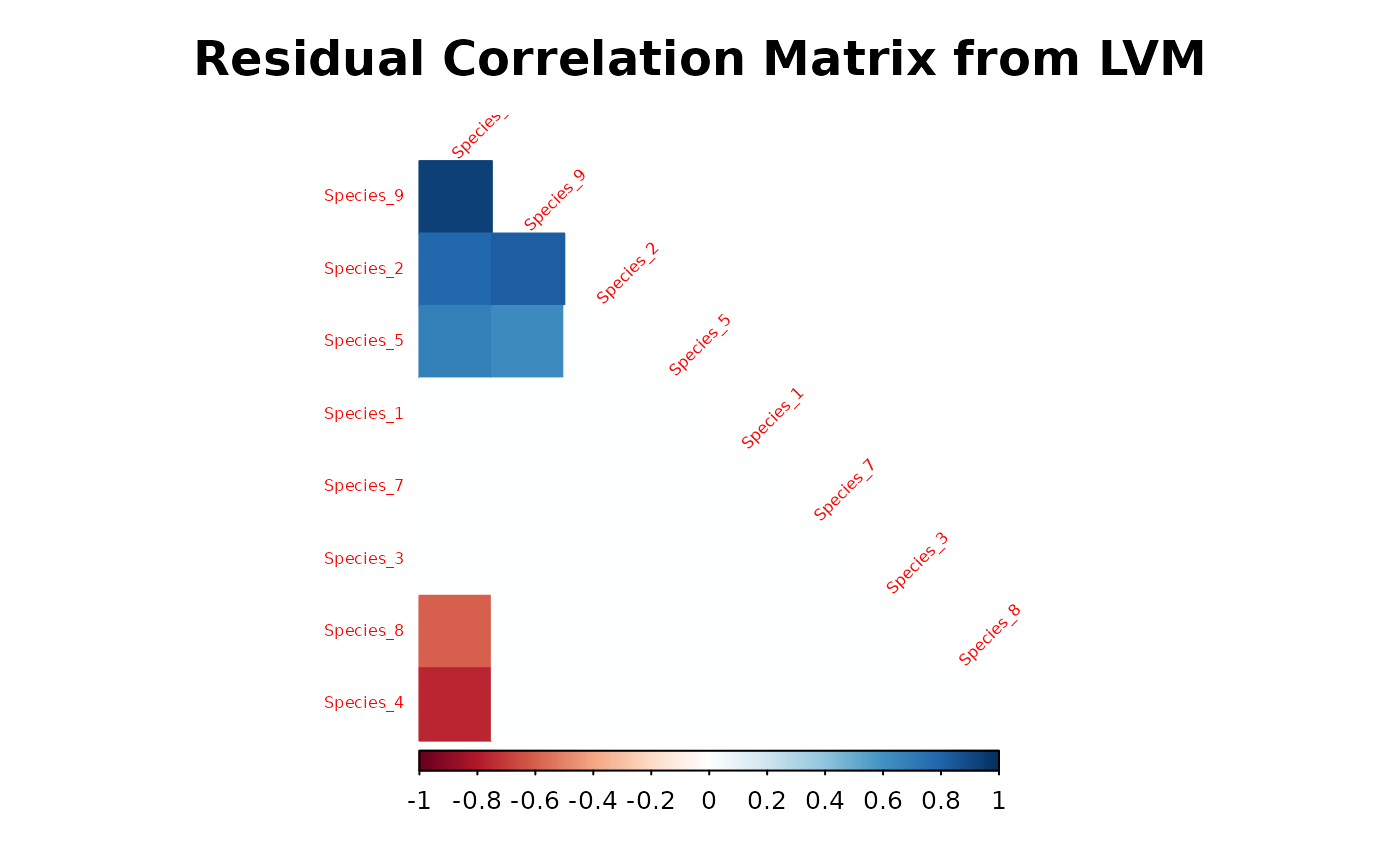Plot the posterior mean estimator of residual correlation matrix reordered by first principal component using corrplot function from the package of the same name.

plot_residual_cor(
mod,
prob = NULL,
title = "Residual Correlation Matrix from LVM",
diag = F,
type = "lower",
method = "color",
mar = c(1, 1, 3, 1),
tl.srt = 45,
tl.cex = 0.5,
...
)

## Arguments

mod

An object of class "jSDM".

prob

A numeric scalar in the interval $$(0,1)$$ giving the target probability coverage of the intervals, by which to determine whether the correlations are "significant". If prob=0.95 is specified only significant correlations, whose $$95\%$$ HPD interval does not contain zero, are represented. Defaults to prob=NULL to represent all correlations significant or not.

title

Character, title of the graph.

diag

Logical, whether display the correlation coefficients on the principal diagonal.

type

Character, "full" (default), "upper" or "lower", display full matrix, lower triangular or upper triangular matrix.

method

Character, the visualization method of correlation matrix to be used. Currently, it supports seven methods, named "circle" (default), "square", "ellipse", "number", "pie", "shade" and "color".

mar

See par

tl.srt

Numeric, for text label string rotation in degrees, see text.

tl.cex

Numeric, for the size of text label (variable names).

...

Further arguments passed to corrplot function

## Value

Displays a reordered correlation matrix

corrplot jSDM-package jSDM_binomial_probit
jSDM_binomial_logit jSDM_poisson_log

## Author

Jeanne Clément <jeanne.clement16@laposte.net>

## Examples

library(jSDM)
# frogs data
data(frogs, package="jSDM")
# Arranging data
PA_frogs <- frogs[,4:12]
# Normalized continuous variables
Env_frogs <- cbind(scale(frogs[,1]),frogs[,2],scale(frogs[,3]))
colnames(Env_frogs) <- colnames(frogs[,1:3])
# Parameter inference
# Increase the number of iterations to reach MCMC convergence
mod<-jSDM_binomial_probit(# Response variable
presence_data = PA_frogs,
# Explanatory variables
site_formula = ~.,
site_data = Env_frogs,
n_latent=2,
site_effect="random",
# Chains
burnin=100,
mcmc=100,
thin=1,
# Starting values
alpha_start=0,
beta_start=0,
lambda_start=0,
W_start=0,
V_alpha=1,
# Priors
shape=0.1, rate=0.1,
mu_beta=0, V_beta=1,
mu_lambda=0, V_lambda=1,
# Various
seed=1234, verbose=1)
#>
#> Running the Gibbs sampler. It may be long, please keep cool :)
#>
#> **********:10.0%
#> **********:20.0%
#> **********:30.0%
#> **********:40.0%
#> **********:50.0%
#> **********:60.0%
#> **********:70.0%
#> **********:80.0%
#> **********:90.0%
#> **********:100.0%
# Representation of residual correlation between species
plot_residual_cor(mod)plot_residual_cor(mod, prob=0.95)